# Matrix formulation of an operator

tanaygupta2000
Homework Statement:
Consider an N-dimensional linear vector space spanned by the ortho-normal basis
states, {|b1>, |b2>, ...., |bN>}. An operator R is given to operate on these basis stats as: R|b(j) = |b(j+1)> for j=1 to (N-1), and R|bN> = |b1>.
Write R in the matrix form in the given basis.
Relevant Equations:
<ψ|R|ψ> = Σ(i) Σ(j) <ψ|bj> <bj|R|bi> <bi|ψ>
where
R operator = <bj|R|bi> matrix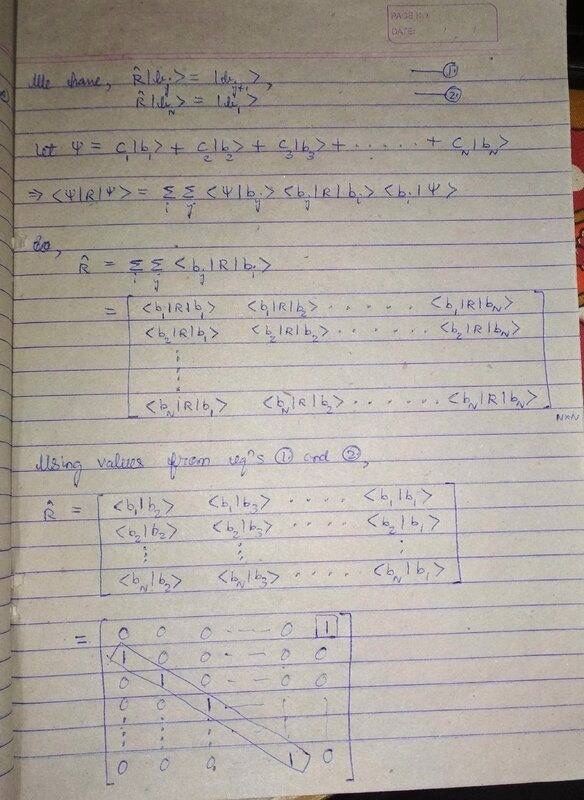I have successfully found the N by N matrix corresponding to the operator R.
But the problem is, whenever I try to operate R on |bj> basis vectors, I am not getting |b(j+1)> as it should be.
Instead, I am getting result as given in the question only by <bj|R = <b(j+1)|

Matrix is not working with kets.

Homework Helper
Gold Member
2022 Award
Homework Statement:: Consider an N-dimensional linear vector space spanned by the ortho-normal basis
states, {|b1>, |b2>, ..., |bN>}. An operator R is given to operate on these basis stats as: R|b(j) = |b(j+1)> for j=1 to (N-1), and R|bN> = |b1>.
Write R in the matrix form in the given basis.
Relevant Equations:: <ψ|R|ψ> = Σ(i) Σ(j) <ψ|bj> <bj|R|bi> <bi|ψ>
where
R operator = <bj|R|bi> matrix

View attachment 278350

I have successfully found the N by N matrix corresponding to the operator R.
But the problem is, whenever I try to operate R on |bj> basis vectors, I am not getting |b(j+1)> as it should be.
Instead, I am getting result as given in the question only by <bj|R = <b(j+1)|

Matrix is not working with kets.
The matrix looks right to me. What do you get for ##R|b_1 \rangle##?

•tanaygupta2000
tanaygupta2000
The matrix looks right to me. What do you get for ##R|b_1 \rangle##?
It is behaving like R|b(j) = |b(j-1)> instead of R|b(j) = |b(j+1)>

Homework Helper
Gold Member
2022 Award
It is behaving like R|b(j) = |b(j-1)> instead of R|b(j) = |b(j+1)>
The question was what is ##R|b_1 \rangle##?

Homework Helper
Gold Member
2022 Award
Hmm! $$|b_1 \rangle = (1, 0, \dots 0)^T$$

tanaygupta2000
Hmm! $$|b_1 \rangle = (1, 0, \dots 0)^T$$
But then R|b1> will be zero?
Because R11 is zero

Homework Helper
Gold Member
2022 Award
But then R|b1> will be zero?
No. It will be ##b_2##.

Homework Helper
Gold Member
2022 Award
Try with ##N = 3##.

•tanaygupta2000
tanaygupta2000
Try with ##N = 3##.
For N=2, R = [0, 0 first row and 1, 0 second row]
Is it right?

Homework Helper
Gold Member
2022 Award
For N=2, R = [0, 0 first row and 1, 0 second row]
Is it right?

tanaygupta2000
For N=3, R = [0, 0, 0; 1, 0, 0; 0, 1, 0]
R|b1> is still 0
since first row is zero

Homework Helper
Gold Member
2022 Award
For N=3, R = [0, 0, 0; 1, 0, 0; 0, 1, 0]
R|b1> is still 0
Well, no. ##R## has a ##1## at the end of the first row.

•tanaygupta2000
tanaygupta2000
Well, no. ##R## has a ##1## at the end of the first row.
Sir the end has <b1|b4>
How it can be 1?

Homework Helper
Gold Member
2022 Award
Sir the end has <b1|b4>
How it can be 1?
There is no ##b_4## if ##N = 3##.

tanaygupta2000
Sir the end has <b1|b4>
How it can be 1?
In accordance with <b1|R|b3>

tanaygupta2000
There is no ##b_4## if ##N = 3##.
So what will be <b1|R|b3>?
I am getting confused

Homework Helper
Gold Member
2022 Award
So what will be <b1|R|b3>?
I am getting confused
I can see that. From your post #5, we have:
$$R(N=3) = [0, 0, 1; 1, 0, 0; 0, 1, 0]$$

•tanaygupta2000
tanaygupta2000
I can see that. From your post #5, we have:
$$R(N=3) = [0, 0, 1; 1, 0, 0; 0, 1, 0]$$
Sir are the last entries of rows II and III belong from the last column of R(N, N)?

tanaygupta2000
I think I am getting ...

Homework Helper
Gold Member
2022 Award
Sir are the last entries of rows II and III belong from the last column of R(N, N)?
If ##N = 3##, then the ##1## at the end is in the 3rd column. ##N## is a variable in this case.

tanaygupta2000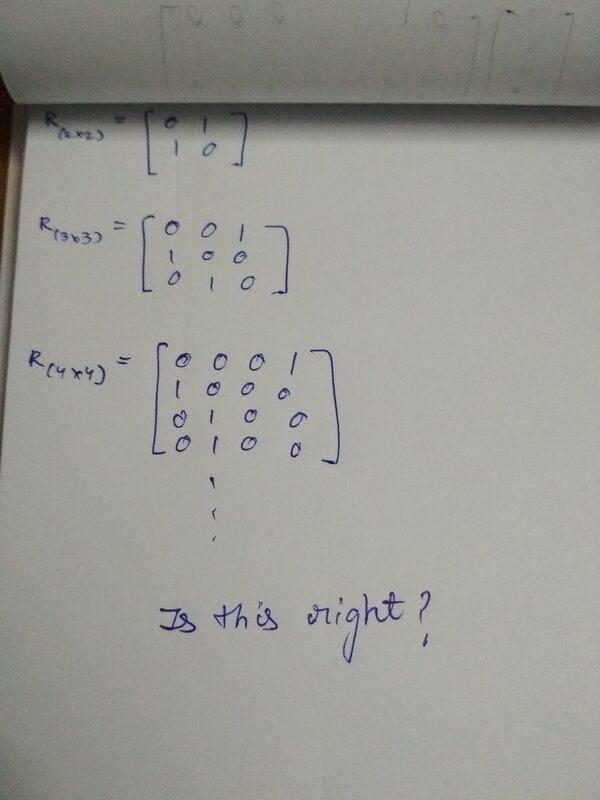•PeroK
Homework Helper
Gold Member
2022 Award
Not exactly, the last row is wrong on ##R_4##!

•tanaygupta2000
tanaygupta2000
Thank You so much !

Homework Helper
Gold Member
2022 Award
Thank You so much !
Okay, but note that I've spotted an error now.

tanaygupta2000
Okay, but note that I've spotted an error now.
Yes sir my mistake it should be 0, 0, 1, 0

Homework Helper
Gold Member
2022 Award
Yes sir my mistake it should be 0, 0, 1, 0
That's right now.

•tanaygupta2000
tanaygupta2000
No. It will be ##b_2##.
Sir please assist me where am I doing mistake.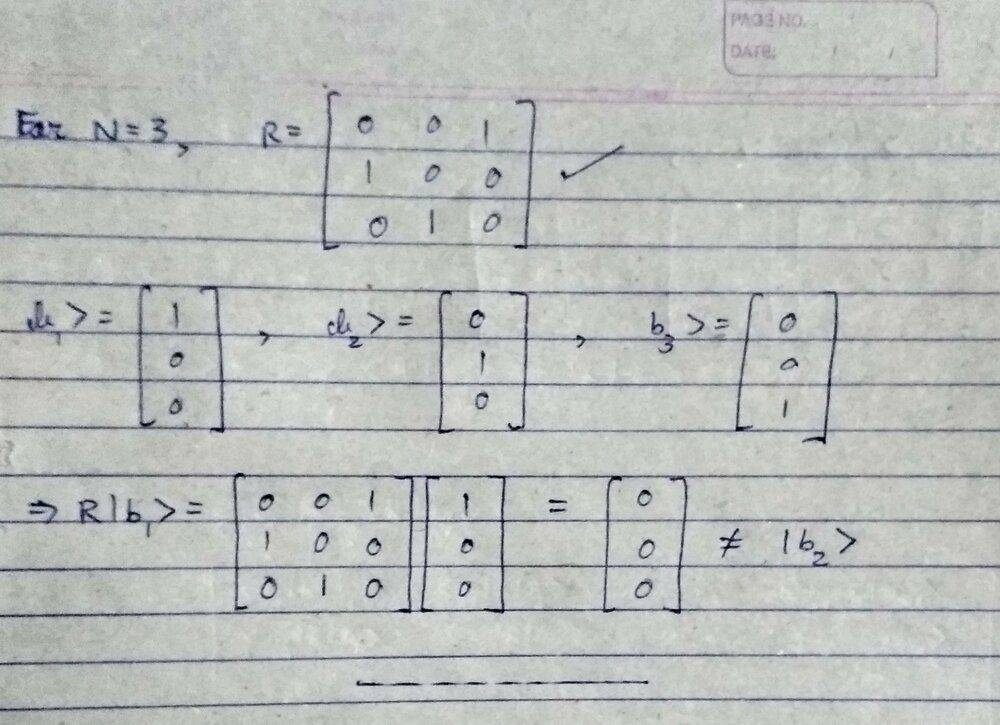tanaygupta2000
In Shankar it is given like this: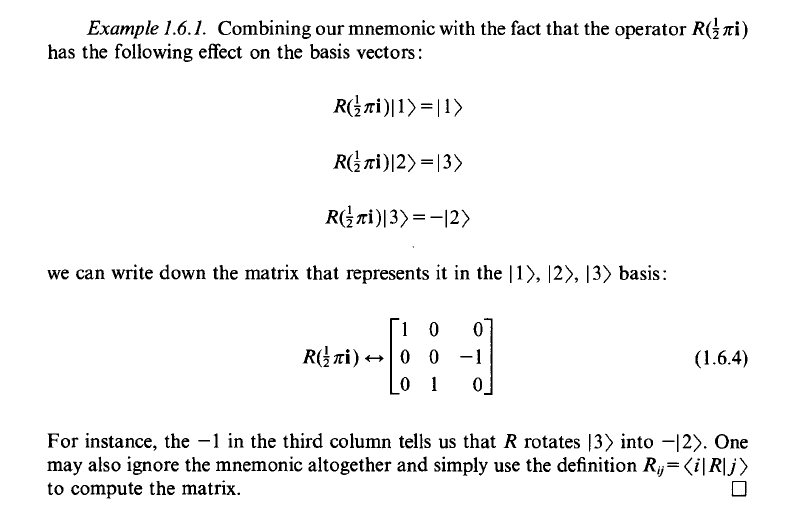But I'm having trouble verifying it mathematically as given in the question.

tanaygupta2000
In Shankar it is given like this:
View attachment 278401

But I'm having trouble verifying it mathematically as given in the question.
I just tried using a hermitian of R since it is behaving like a rotation matrix and I got ... is this good?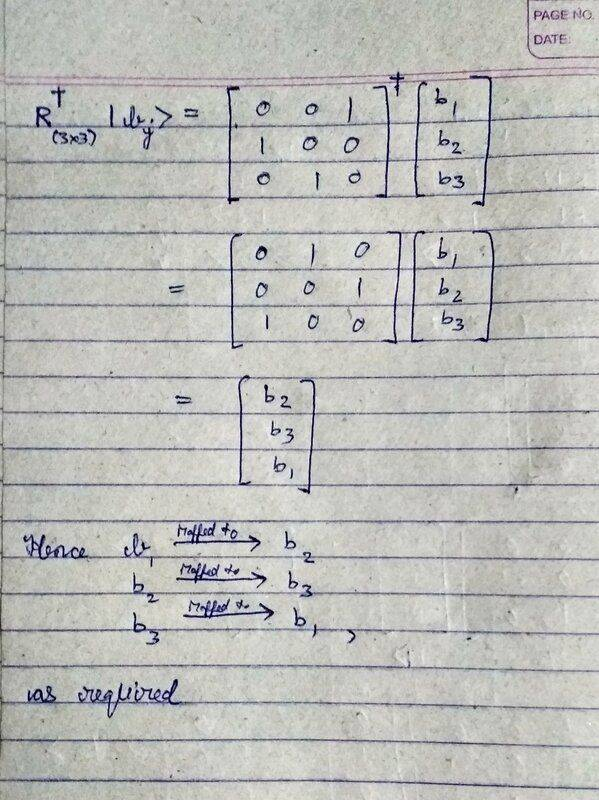Homework Helper
Gold Member
2022 Award
Sir please assist me where am I doing mistake.View attachment 278400
That's simply wrong. I can't imagine what process you're using for matrix multiplication of a vector, but it's not the right one.

Homework Helper
Gold Member
2022 Award
I just tried using a hermitian of R since it is behaving like a rotation matrix and I got ... is this good?View attachment 278403
You're matrix multiplication is correct, but you have the wrong idea about what a basis vector is.

tanaygupta2000
You're matrix multiplication is correct, but you have the wrong idea about what a basis vector is.
You told it is b1> = transpose(1, 0, 0, ...,)

Homework Helper
Gold Member
2022 Award
You told it is b1> = transpose(1, 0, 0, ...,)
Which is right. You're confusing it with the first component of a vector.